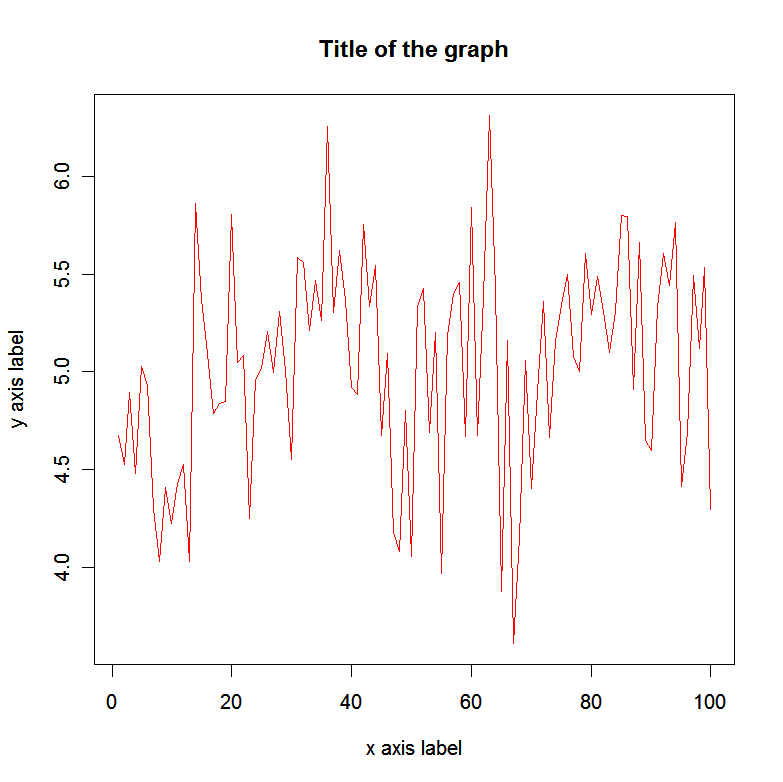## Friday, August 17, 2012

### If you cannot receive new mail in Thunderbird - compact your folders

In Thunderbird if you get the message that your inbox is full and you cannot receive any more new emails you need to compact your folders.
Don't try to delete your mails, you can delete complete inbox and still get the same message.
Go to File/Compact folders

## Sunday, August 5, 2012

### How to put multiple plots in one overall graph in R

To put multiple plots in one overall graph use par function to separate the graph in (x,y) fields.
For example:

> par (mfrow=c(2,2))
> plot(sort(medalcount\$Gold), col=6, lwd=1, type="o")
> plot(sort(medalcount\$Silver), col=6, lwd=1, type="o")
> plot(sort(medalcount\$Bronze), col=6, lwd=1, type="o")
> plot(sort(medalcount\$Total), col=6, lwd=1, type="o")

gave me the following output:

## Saturday, August 4, 2012

### How to restore username and password autofill option in Chrome

If you have imported your passwords to Chrome you may notice that on some websites they will not appear autofilled. This most probably happened if the URL in question is not recognized by Chrome for some reason, but still it being present in the list will prevent Chrome to offer to save password when you enter it.
Go to:
Settings/Show advanced settings
Privacy/Manage saved passwords
Find the website and delete the password/site.
Type the correct URL and username/password, when Chrome asks to save it accept.
The password should appear autofilled next time you visit the site.

## Friday, August 3, 2012

### How to add line to a plot in R

Lets say you have a scatter plot and want to make a linear regression line.

For example we will create two variables and plot them in a scatter plot.
a <- c(1:100)
b <- rnorm(100, 5, 0.5)
plot(a,b)

For linear regression line type:

If you want to add custom line you could also use abline command, with the parameters a=intersection with y axes and b=slope of the curve (hence the name of the command abline)
For example:
abline(5,0, col=14, lwd=2)

### How to add data points on plots in R

In R if you want to add a custom data point on the graph, e.g on the xy position (40,6), with the color 40 ("burlywood3"), and with the line width 3, type:
points(40,6, col=14, lwd=3)

If we follow up the graph made in the previous post:This would be the resulting graph:If we want to add multiple points type e.g. the following:
points(c(10,20,30,40,50,60,70,80,90),c(4,4,4,5,5,5,6,6,6), col=14, lwd=3)

If you want to connect these points into a line:
points(c(10,20,30,40,50,60,70,80,90),c(4,4,4,5,5,5,6,6,6), col=14, lwd=3, type="l")

### How to make simple scatter plots in R

How to make a simple scatter plot in R:

For example we will create two variables and plot them in a scatter plot.
a <- c(1:100)
b <- rnorm(100, 5, 0.5)

plot(a,b)If we want to make a line instead of dots:
plot(a,b, type="l")To change the title, axis labels, and color of the line type the following:
plot(a,b, type="l",main="Title of the graph", xlab="x axis label", ylab="y axis label", col=634)
For the list of colors type:
colors()